SNOSAW8E May   2008  – September 2015

PRODUCTION DATA.

1. Features
2. Applications
3. Description
4. Revision History
5. Description continued
6. Pin Configuration and Functions
7. Specifications
8. Detailed Description
1. 8.1 Overview
2. 8.2 Functional Block Diagram
3. 8.3 Feature Description
4. 8.4 Device Functional Modes
9. Application and Implementation
1. 9.1 Application Information
2. 9.2 Typical Application
10. 10Power Supply Recommendations
11. 11Layout
12. 12Device and Documentation Support
13. 13Mechanical, Packaging, and Orderable Information

• DBV|5

## 8 Detailed Description

### 8.1 Overview

The LM732xx devices are rail-to-rail input and output amplifiers with wide operating voltages and high-output currents. The LM732xx family is efficient, achieving 18-V/µs slew rate and 20-MHz unity gain bandwidth while requiring only 1 mA of supply current per op amp. The LM732xx device performance is fully specified for operation at 2.7 V, ±5 V and ±15 V.

The LM732xx devices are designed to drive unlimited capacitive loads without oscillations. All LM7321x and LM7322x parts are tested at −40°C, 125°C, and 25°C, with modern automatic test equipment. High performance from −40°C to 125°C, detailed specifications, and extensive testing makes them suitable for industrial, automotive, and communications applications.

Greater than rail-to-rail input common-mode voltage range with 50 dB of common-mode rejection across this wide voltage range, allows both high-side and low-side sensing. Most device parameters are insensitive to power supply voltage, and this makes the parts easier to use where supply voltage may vary, such as automotive electrical systems and battery-powered equipment. These amplifiers have true rail-to-rail output and can supply a respectable amount of current (15 mA) with minimal head room from either rail (300 mV) at low distortion (0.05% THD+Noise).

### 8.2 Functional Block Diagram### 8.3 Feature Description

#### 8.3.1 Output Short Circuit Current and Dissipation Issues

The LM732xx output stage is designed for maximum output current capability. Even though momentary output shorts to ground and either supply can be tolerated at all operating voltages, longer lasting short conditions can cause the junction temperature to rise beyond the absolute maximum rating of the device, especially at higher supply voltage conditions. Below supply voltage of 6 V, the output short circuit condition can be tolerated indefinitely.

With the op amp tied to a load, the device power dissipation consists of the quiescent power due to the supply current flow into the device, in addition to power dissipation due to the load current. The load portion of the power itself could include an average value (due to a DC load current) and an AC component. DC load current would flow if there is an output voltage offset, or the output AC average current is non-zero, or if the op amp operates in a single supply application where the output is maintained somewhere in the range of linear operation.

Therefore,

Equation 1. PTOTAL = PQ + PDC + PAC

The Op Amp Quiescent Power Dissipation is calculated as:

Equation 2. PQ = IS × VS

where

• IS: Supply Current
• VS: Total Supply Voltage (V+ − V)

The DC Load Power is calculated as:

Equation 3. PDC = IO × (Vr - Vo)

where

• VO: Average Output Voltage
• Vr: V+ for sourcing and V for sinking current

The AC Load Power is calculated as PAC = See Table 1.

Table 1 shows the maximum AC component of the load power dissipated by the op amp for standard Sinusoidal, Triangular, and Square Waveforms:

### Table 1. Normalized AC Power Dissipated in the Output Stage for Standard Waveforms

PAC (W.Ω/V2)
Sinusoidal Triangular Square
50.7 × 10−3 46.9 × 10−3 62.5 × 10−3

The table entries are normalized to VS2/RL. To figure out the AC load current component of power dissipation, simply multiply the table entry corresponding to the output waveform by the factor VS2/RL. For example, with ±12-V supplies, a 600-Ω load, and triangular waveform power dissipation in the output stage is calculated as:

Equation 4. PAC = (46.9 × 10−3) × (242/600) = 45.0 mW

The maximum power dissipation allowed at a certain temperature is a function of maximum die junction temperature (TJ(MAX)) allowed, ambient temperature TA, and package thermal resistance from junction to ambient, θJA.

Equation 5.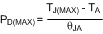For the LM732xx, the maximum junction temperature allowed is 150°C at which no power dissipation is allowed. The power capability at 25°C is given by the following calculations:

For VSSOP package:

Equation 6.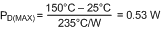For SOIC package:

Equation 7.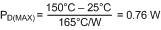Similarly, the power capability at 125°C is given by:

For VSSOP package:

Equation 8.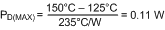For SOIC package:

Equation 9.Figure 58 shows the power capability vs. temperature for VSSOP and SOIC packages. The area under the maximum thermal capability line is the operating area for the device. When the device works in the operating area where PTOTAL is less than PD(MAX), the device junction temperature will remain below 150°C. If the intersection of ambient temperature and package power is above the maximum thermal capability line, the junction temperature will exceed 150°C and this should be strictly prohibited.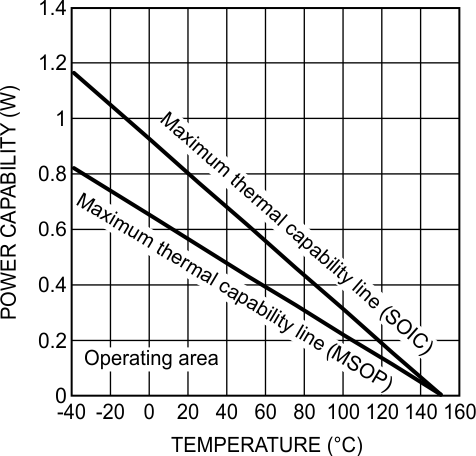Figure 58. Power Capability vs. Temperature

When high power is required and ambient temperature can't be reduced, providing air flow is an effective approach to reduce thermal resistance therefore to improve power capability.

#### 8.3.2 Estimating the Output Voltage Swing

It is important to keep in mind that the steady-state output current will be less than the current available when there is an input overdrive present. For steady-state conditions, the Output Voltage vs. Output Current plot (Typical Characteristics section) can be used to predict the output swing. Figure 59 and Figure 60 show this performance along with several load lines corresponding to loads tied between the output and ground. In each cases, the intersection of the device plot at the appropriate temperature with the load line would be the typical output swing possible for that load. For example, a 1-kΩ load can accommodate an output swing to within 250 mV of V and to 330 mV of V+ (VS = ±15 V) corresponding to a typical 29.3 VPP unclipped swing.Figure 59. Output Sourcing Characteristics With Load Lines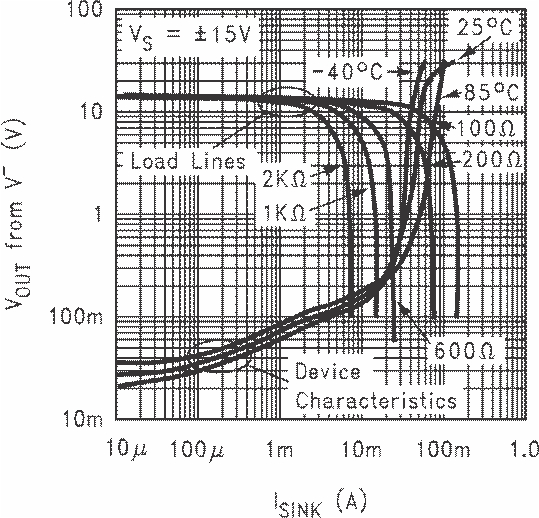Figure 60. Output Sinking Characteristics With Load Lines

### 8.4 Device Functional Modes

The LM732xx are specifically designed to drive unlimited capacitive loads without oscillations as shown in Figure 61.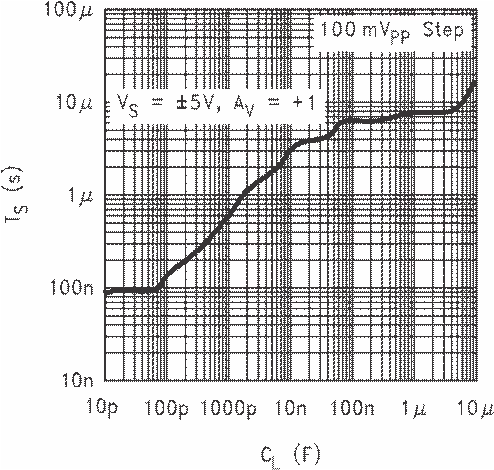Figure 61. ±5% Settling Time vs. Capacitive Load

In addition, the output current handling capability of the device allows for good slewing characteristics even with large capacitive loads as shown in Figure 62 and Figure 63.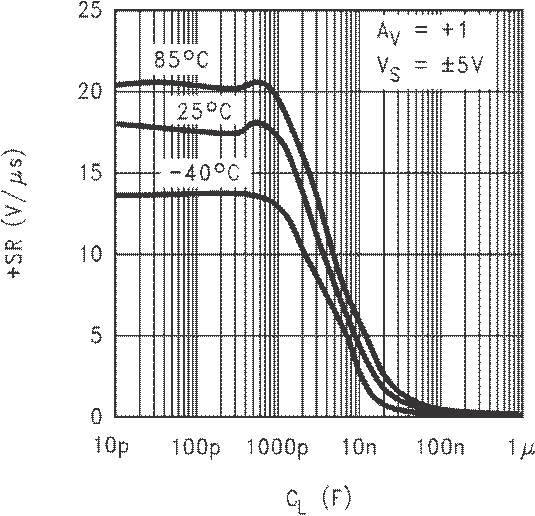Figure 62. +SR vs. Capacitive LoadFigure 63. −SR vs. Capacitive Load

The combination of these features is ideal for applications such as TFT flat panel buffers, A/D converter input amplifiers, and so forth.

However, as in most op amps, addition of a series isolation resistor between the op amp and the capacitive load improves the settling and overshoot performance.

Output current drive is an important parameter when driving capacitive loads. This parameter will determine how fast the output voltage can change. Referring to the Slew Rate vs. Capacitive Load Plots (Typical Characteristics section), two distinct regions can be identified. Below about 10,000 pF, the output Slew Rate is solely determined by the compensation capacitor value of the op amp and available current into that capacitor. Beyond 10 nF, the Slew Rate is determined by the available output current of the op amp.

NOTE

Because of the lower output sourcing current compared to the sinking one, the Slew Rate limit under heavy capacitive loading is determined by the positive transitions.

An estimate of positive and negative slew rates for loads larger than 100 nF can be made by dividing the short circuit current value by the capacitor.

For the LM732xx, the available output current increases with the input overdrive. Referring to Figure 64 and Figure 65, it can be seen that both sourcing and sinking short circuit current increase as input overdrive increases. In a closed-loop amplifier configuration, during transient conditions while the fed back output has not quite caught up with the input, there will be an overdrive imposed on the input allowing more output current than would normally be available under steady-state condition. Because of this feature, the output stage quiescent current of the op amp can be kept to a minimum, thereby reducing power consumption, while enabling the device to deliver large output current when the need arises (such as during transients).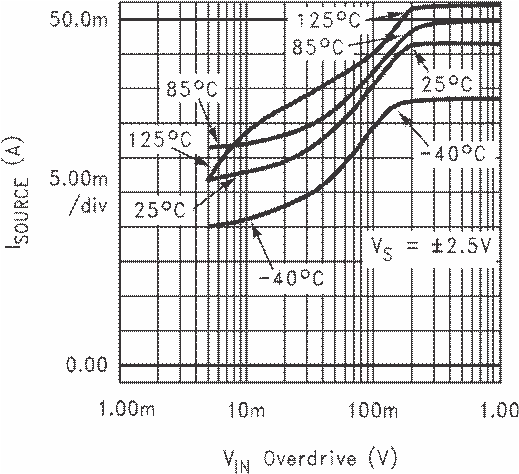Figure 64. Output Short Circuit Sourcing Current vs. Input Overdrive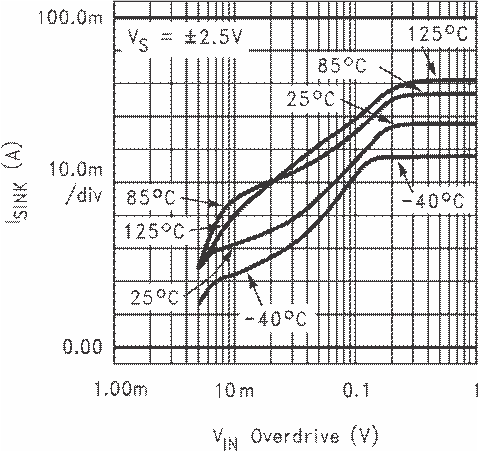Figure 65. Output Short Circuit Sinking Current vs. Input Overdrive

Figure 66 shows the output voltage, output current, and the resulting input overdrive with the device set for AV = +1 and the input tied to a 1-VPP step function driving a 47-nF capacitor. As can be seen, during the output transition, the input overdrive reaches 1-V peak and is more than enough to cause the output current to increase to its maximum value (see Figure 64 and Figure 65 plots).

NOTE

Because of the larger output sinking current compared to the sourcing one, the output negative transition is faster than the positive one.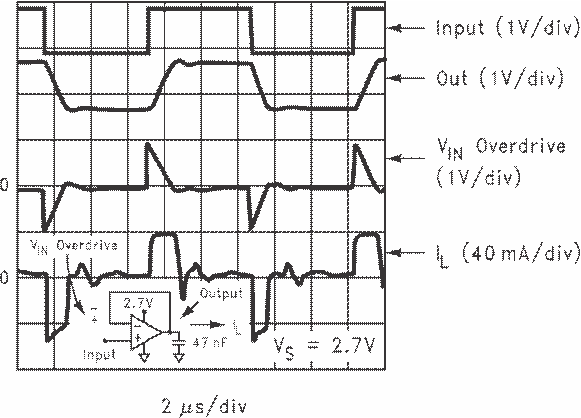Figure 66. Buffer Amplifier Scope Photo